# Pytim: Quick Tour¶

Pytim is a package based on MDAnalysis for the identification and analysis of surface molecules in configuration files or in trajectories from molecular dynamics simulations.

Although MDAnalysis is needed to perform the interfacial analysis, you can also use Pytim on top MDTraj or directly online from a simulation performed using OpenMM. For more information see the Tutorials.

## Basic Example¶

This is a basic example that shows how easy is to use the `ITIM` class for planar interfaces:

```import MDAnalysis as mda
import pytim
from   pytim.datafiles import *

# load an example configuration file
u         = mda.Universe(WATER_GRO)

# initialize the interface, this has to be called only once
# before iterating over the trajectory!
interface = pytim.ITIM(u)

# write a pdb with the information on the surface layers
interface.writepdb('layers.pdb',centered='middle')

# access the atoms in the layers as an MDAnalysis' AtomGroups
upper_layer = interface.layers[0,0]
```

The above lines are doing the following:

1. Import the modules of MDAanalysis, pytim, and some example datafiles, then initialize the MDAnalysis universe in the usual way, using as an input file one of the structures provided by the package, in this case a water/vapor interface in a gromos file format (the variable WATER_GRO is made available by the pytim.datafiles module)
2. The interfacial analysis is then initialized using the `ITIM` class, and the molecular layers are calculated automatically.
3. The whole configuration is saved to a pdb for graphical inspection using `writepdb()` (with surface atoms having a beta factor equal to the numer of the layer they belong to).
4. The groups of atoms corresponding to different layers can be extracted using `layers()`

The result of the calculation can be seen in the following picture, where surface oxygen atoms are highlighted in blue.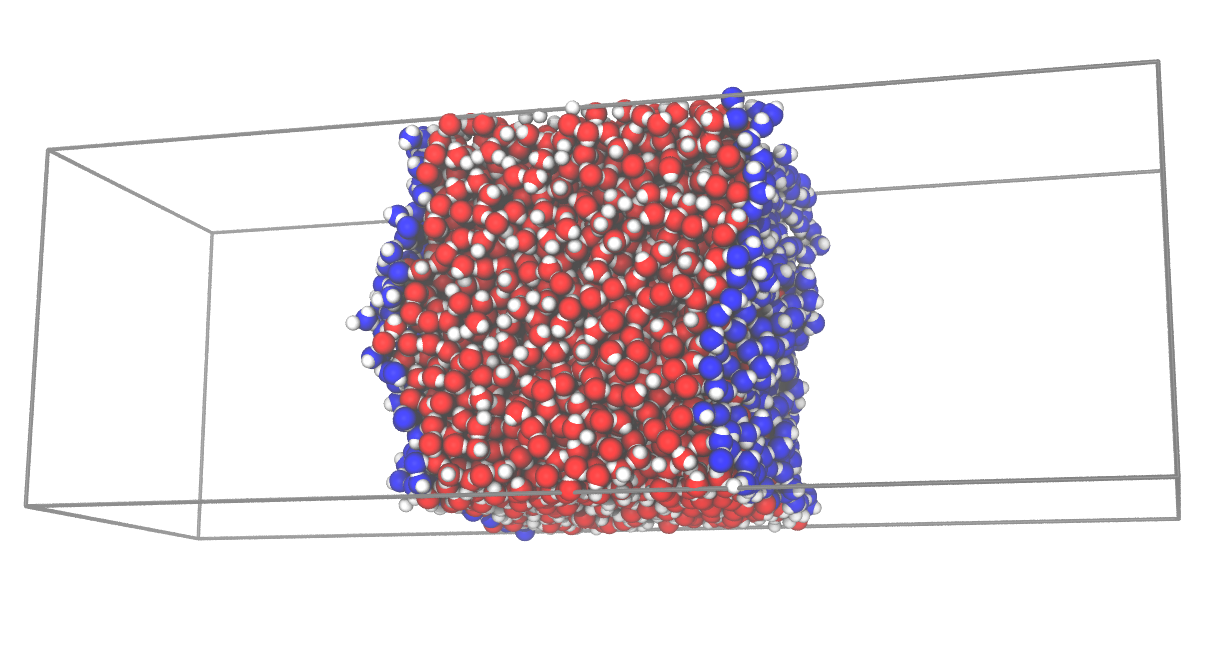This is a very basic example, and more are given below, in the Tutorials, and in the documentation pages of the modules.

## Non-planar interfaces¶

### GITIM¶

One of the possibilities is to use `GITIM` to identify surface atoms in a conceptually similar way to `ITIM`. We make here the example of multiple solvation layers around glucose:

```import MDAnalysis as mda
import pytim
from   pytim.datafiles import GLUCOSE_PDB

u       = mda.Universe(GLUCOSE_PDB)
solvent = u.select_atoms('name OW')
glc     = u.atoms - solvent.residues.atoms

# alpha is the probe-sphere radius
inter = pytim.GITIM(u, group=solvent, max_layers=3, alpha=2)

for i in [0,1,2]:
print "Layer "+str(i),repr(inter.layers[i])

Layer 0 <AtomGroup with 54 atoms>
Layer 1 <AtomGroup with 117 atoms>
Layer 2 <AtomGroup with 216 atoms>
```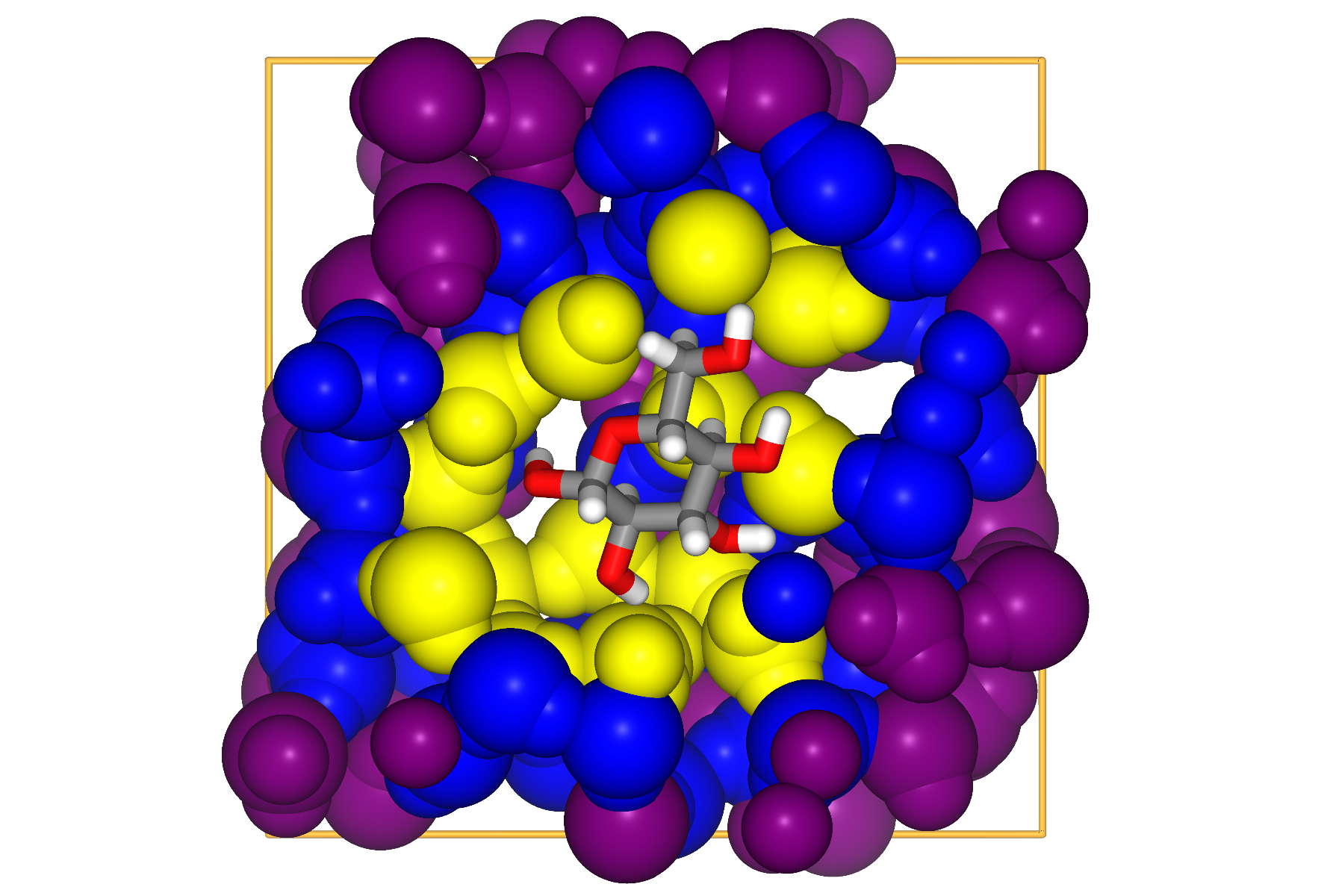### Willard-Chandler¶

If one is not directly interested in interfacial atoms, but in the location of the instantaneous, continuous surface, it is possible to use the method of Willard and Chandler. Options for the output are the wavefront `obj`, `cube` and `vtk` formats, the last two being able to carry also the information about the atomic positions, besides the surface. The formats can be read by Paraview.

```import MDAnalysis as mda
import pytim
from pytim.datafiles import MICELLE_PDB
import nglview

u = mda.Universe(MICELLE_PDB)
g = u.select_atoms('resname DPC')
# In the WillardChandler module, `alpha` is the Gaussian width  of the kernel
# and `mesh` is the grid size where the continuum surface is sampled
interface = pytim.WillardChandler(u, group=g, mesh=1.5, alpha=3.0)
# particles are written using the option `group`
interface.writecube('data.cube', group = g )
```## Molecular vs Atomic¶

By default methods like `ITIM` or `GITIM` use the `molecular=True` option, meaning that whenever an atom is identified as interfacial, all other atoms in the same residue will be tagged as interfacial. This is usually the appropriate option for small molecular liquids, especially if successive layers are going to be analyzed. Taking the example of water, if all atoms are passed to, say, `ITIM`, and `molecular=False`, the first layer will be composed only of oxygen atoms (the hydrogen atoms being located within the radius of oxygen). As a consequence, the second layer would be composed mostly of hydrogen atoms, and so on. For larger molecules like in the case of lipids, instead, it is more informative to look at the location of different atoms along the surface normal, therefore the `molecular=False` option is advisable, otherwise, as in the case of a small micelle, all atoms in the lipids would be tagged as interfacial.

```import MDAnalysis as mda
import pytim
from pytim.datafiles import MICELLE_PDB
u = mda.Universe(MICELLE_PDB)
g = u.select_atoms('resname DPC')
# pass the `molecular=False` option to identify surface atoms instead of molecules
inter = pytim.GITIM(u,group=g, molecular=False)
```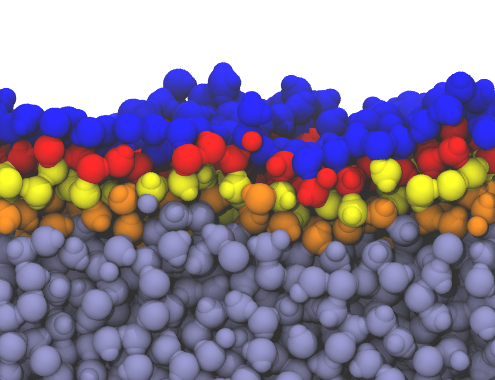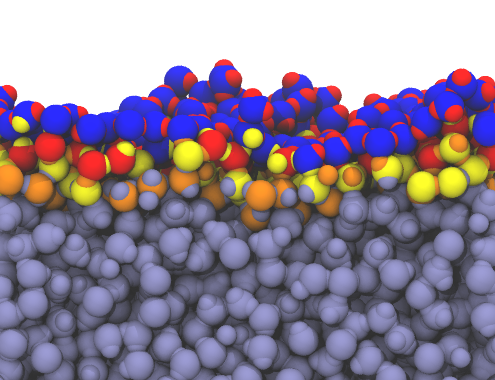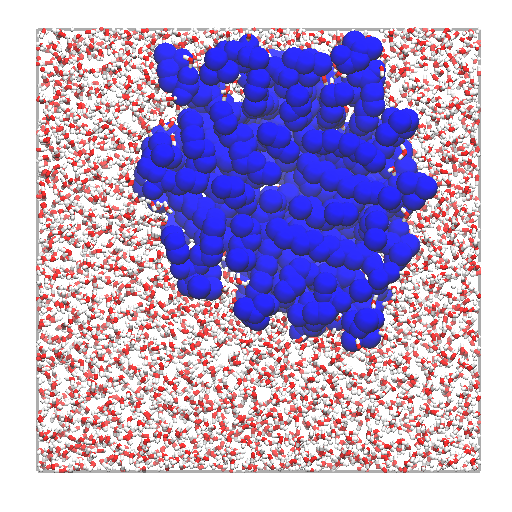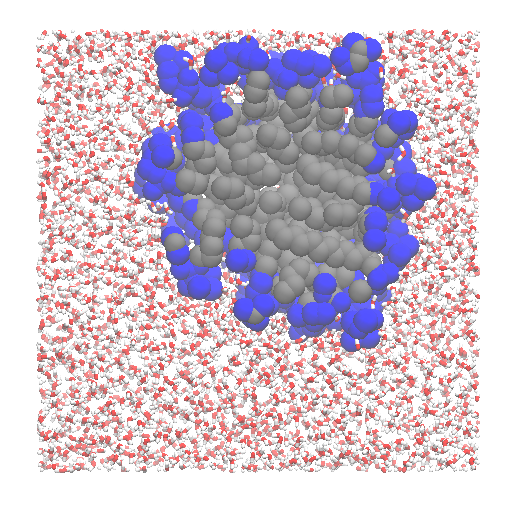Left: layers of interfacial water and micelle (section cut) using `molecular=True`. Right: same using `molecular=False` blue: 1st layer; red: 2nd layer ; yellow: 3rd layer ; orange: 4th layer

## Filtering vapour molecules¶

Pytim offers the option to identify the relevant phases before proceeding to the surface identification. This is a necessary step, for example, if the vapour phase of a water/vapour interface is not empty, or if a two-components system has non-negligible miscibilities.

In order to filter out molecules in the vapour (or in the opposite) phase, pytim relies on different clustering schemes, where the system is partitioned in a set of atoms belonging to the largest cluster, the remaining atoms belonging to the smaller clusters. The available criteria for building the clusters are

1. A simple cutoff criterion of connectivity
2. A local density based clustering criterion (DBSCAN)
In order to use the simple cutoff criterion, it is enough to pass the cluster cutoff to the
`ITIM` or `GITIM` classes, for example:
```import MDAnalysis as mda
import pytim
from pytim.datafiles import WATER_550K_GRO

u = mda.Universe(WATER_550K_GRO)
# 3.5 Angstrom is the approx location of the oxygen g(r) minimum
inter = pytim.ITIM(u,cluster_cut=3.5)
```

At high temperatures, as in this case, using the `cluster_cut` option solves the problem with the molecules in the vapour phase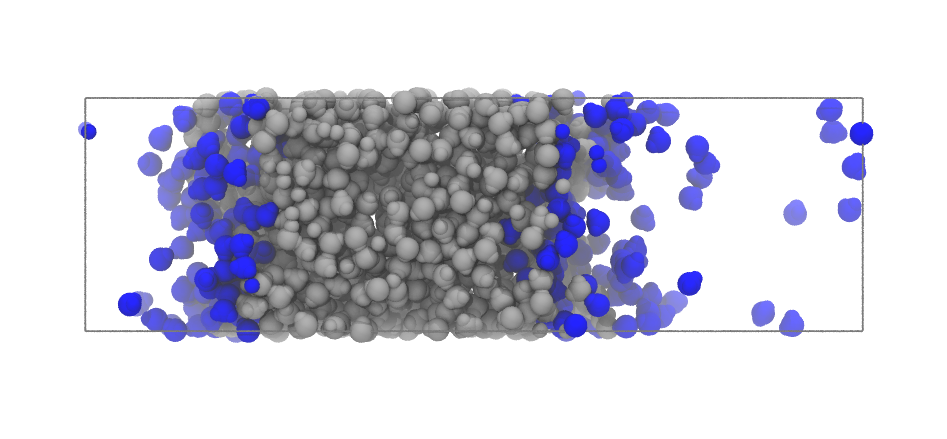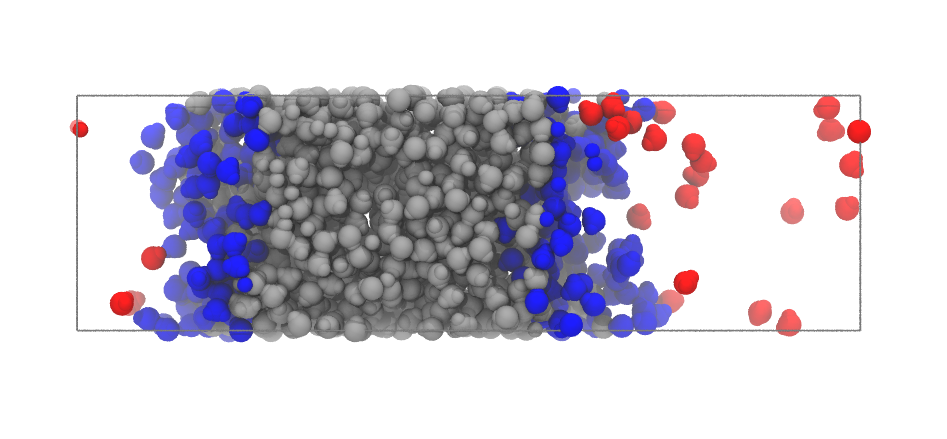Left: Interfacial molecules identified using `ITIM` and no clustering pre-filtering. Right: same system using `cluster_cut=3.5` (blue: first layer; red: vapour phase)

In some cases, the density of the vapour (or opposite) phase is so high, that using any reasonable cutoff, the molecules are percolating the simulation box. In this case, it is advisable to switch to a density based cluster approach. Pytim uses the DBSCAN algorithm, with, in addition, an automated procedure to determine which density should be used to discriminate between liquid and vapour (or high-concentration/low-concentration) regions. An example is a binary mixture of ionic liquids and benzene, which have, respectively, a low and high mutual miscibility.

```import MDAnalysis as mda
import pytim
from   pytim.datafiles import ILBENZENE_GRO

u = mda.Universe(ILBENZENE_GRO)
# LIG is benzene
g = u.select_atoms('resname LIG')
# 1. To switch from the simple clustering scheme to DBSCAN, set the `cluster_threshold_density`
# 2. To estimate correctly the local density, use a larger cutoff than that of the simple clustering
# 3. With `cluster_threshold_density='auto'`, the threshold density is estimated by pytim
inter  = pytim.ITIM(u,group=g,cluster_cut=10.,cluster_threshold_density='auto',alpha=1.5)
```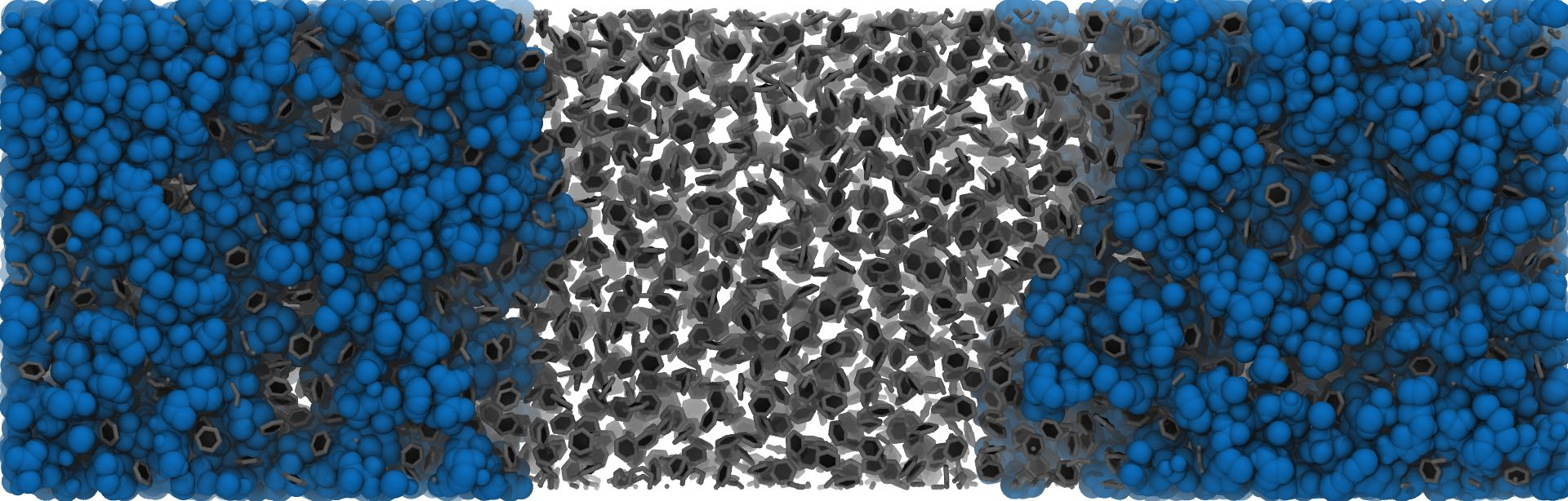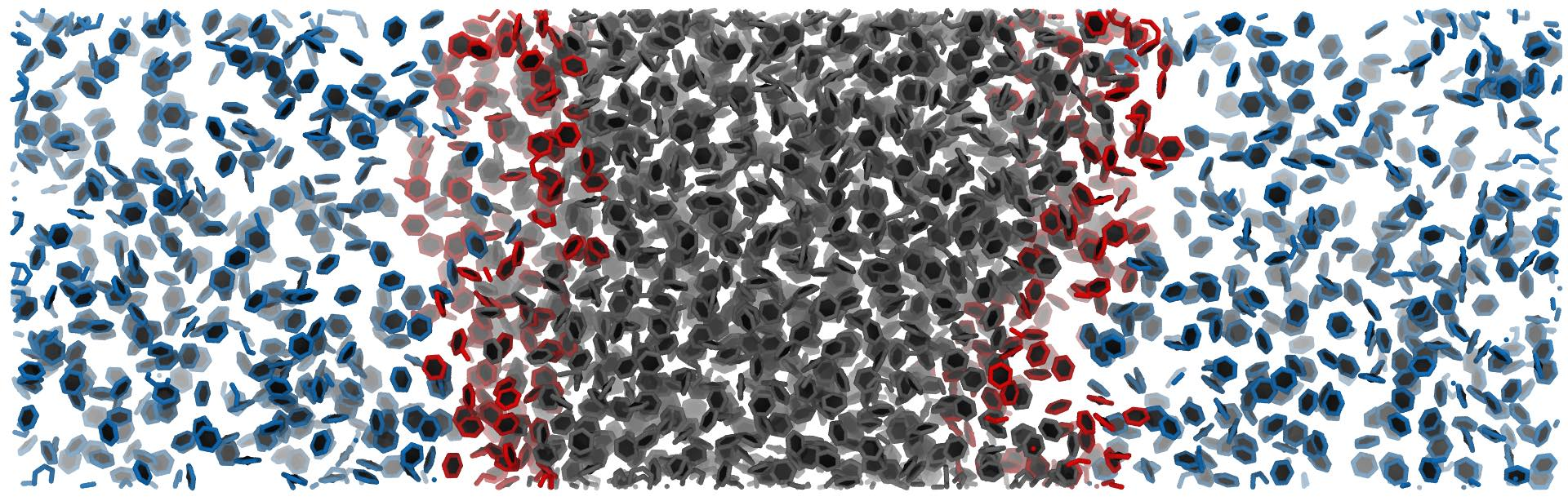Left: the ionic-liquid / benzene mixture, all molecule shown, including the ionic liquid (spheres) and benzene (sticks) Right: benzene phases/interface determined using DBSCAN (options `cluster_cut=10.` and `cluster_threshold_density='auto'` (blue: low-concentration phase; gray: high-concentration phase ; red: interfacial benzene rings.